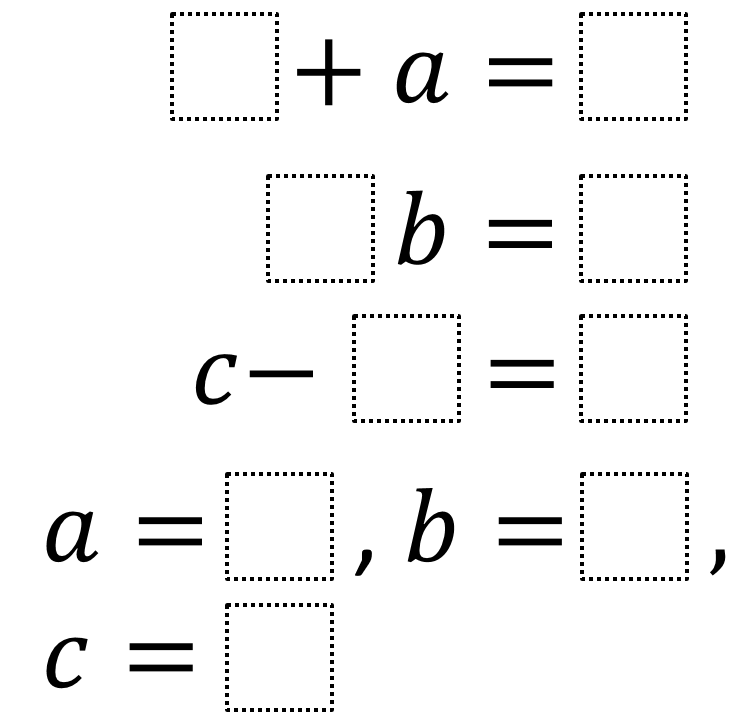Home > Grade 6 > Expressions & Equations > Solving One-Step Equations

# Solving One-Step Equations

Directions: Using the digits 1 to 9 at most one time each, fill in the boxes to make each equation true.### Hint

How does choosing the coefficient or constant affect the variable’s value?
What is the largest possible value that the coefficient of b can be?

### Answer

One answer from Barb Everhart is:
4 + a = 9
3 * b = 6
c – 7 = 1
a = 5, b = 2, c = 8

There are likely other solutions as well.

Source: Robert Kaplinsky

## Subtracting Multi-Decimals

Directions: Use the digits 1 to 9, at most one time each, to fill in …

### 4 comments

1.This answer does not seem to fit the problem (please let me know if I am missing something).

The answer I got was:
4 + a = 9
3 * b = 6
c – 7 = 1
a = 5, b = 2, c = 8

I am using this problem in PD tomorrow, so please let me know if there is an error in my thinking. 🙂

•Thanks Barb. I’m not sure how that answer got switched into this problem. I can’t remember what answer I originally got. Your’s definitely works though. Probably others too.

2.Another student came up with

1+a=8
3b=6
c-4=5
a=7, b=2, c=9

3.The thought process in this question reminds me of Ken Ken Puzzles. These can be played on-line or you can receive an weekly email with printable puzzles. http://www.kenkenpuzzle.com/teachers/classroom

– I do not work for Ken Ken…just love them.